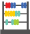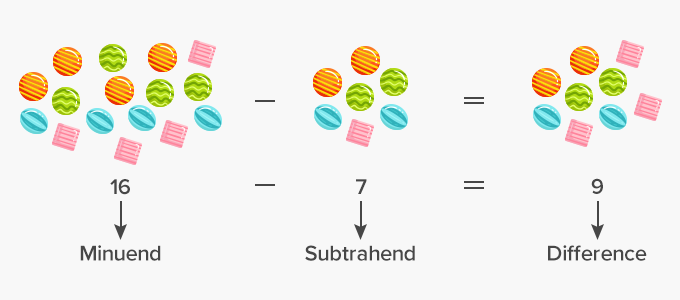# Subtract - Definition with Examples

The Complete K-5 Math Learning Program Built for Your Child

• 30 Million Kids

Loved by kids and parent worldwide

• 50,000 Schools

Trusted by teachers across schools

• Comprehensive Curriculum

Aligned to Common Core

##Let's learn!

What is to subtract?
In math, to subtract means to take away from a group or a number of things.
When we subtract, the number of things in the group reduce or become less.

The minuend, subtrahend and difference are parts of a subtraction problem.
In the subtraction problem, 7 – 3 = 4, the number 7 is the minuend, the number 3 is the subtrahend and the number 4 is the difference

Here’s another example of a subtraction problem.Fun Facts Subtraction is just the opposite of addition. Also, every addition problem can be rewritten as a subtraction problem.

##Let's sing!

Eight little blue birds sitting on a tree,
Five flew away, and then there were three.

##Let's do it!

Instead of handing out math subtraction worksheets to your children, play the number plate game with them while driving them to school or for classes. Ask them to subtract the first, second or third digit in the number plate of the car ahead from the biggest digit in it.  Ask them to be quick in answering.

##Related math vocabulary

Won Numerous Awards & Honors